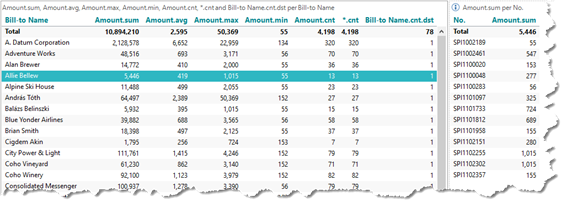# Aggregation types

In the screenshot below, a number of different measure types have been added to the crosstab together with the Bill-to Name dimension. Furthermore, to the right, the cross tab based on the No. dimension will in fact show the individual transactions behind the aggregated data – in this case for the selected Bill-to Name, Allie Bellew.Aggregation type columns explained:

• Bill-to Name: This is a standard dimension that has been picked to be displayed along the vertical axis of the crosstab.
• sum: This is probably the most commonly used measure type. For Allie Bellew, we can see that the total / summarized / aggregated value is 5,446.
• avg: This is the average value across all transactions. For Allie Bellew, the average value is 419, which by the way is equal to Amount.sum divided by *.cnt (5,446 / 13).
• max: This is the maximum value across all transactions.
• min: This is the minimum value across all transactions.
• cnt: This is the distinct count of unique Amount values. For Allie Bellew it is 11. Notice in the table to the right that the values ‘155’ and 1,015’ is represented twice each. This is why the Amount.cnt can be less than the total number of transactions (*.cnt = 13).
• *.cnt: Counts the total number of transactions for each dimension member. Allie Bellew appear in 13 transactions.
• Bill-to Name.cnt: Again, this is a distinct count, so unique appearances of Allie Bellew will only count as 1 – as it will with any other customers in this crosstab. From the Total row, however, we may see that we have in total 78 distinct customers.